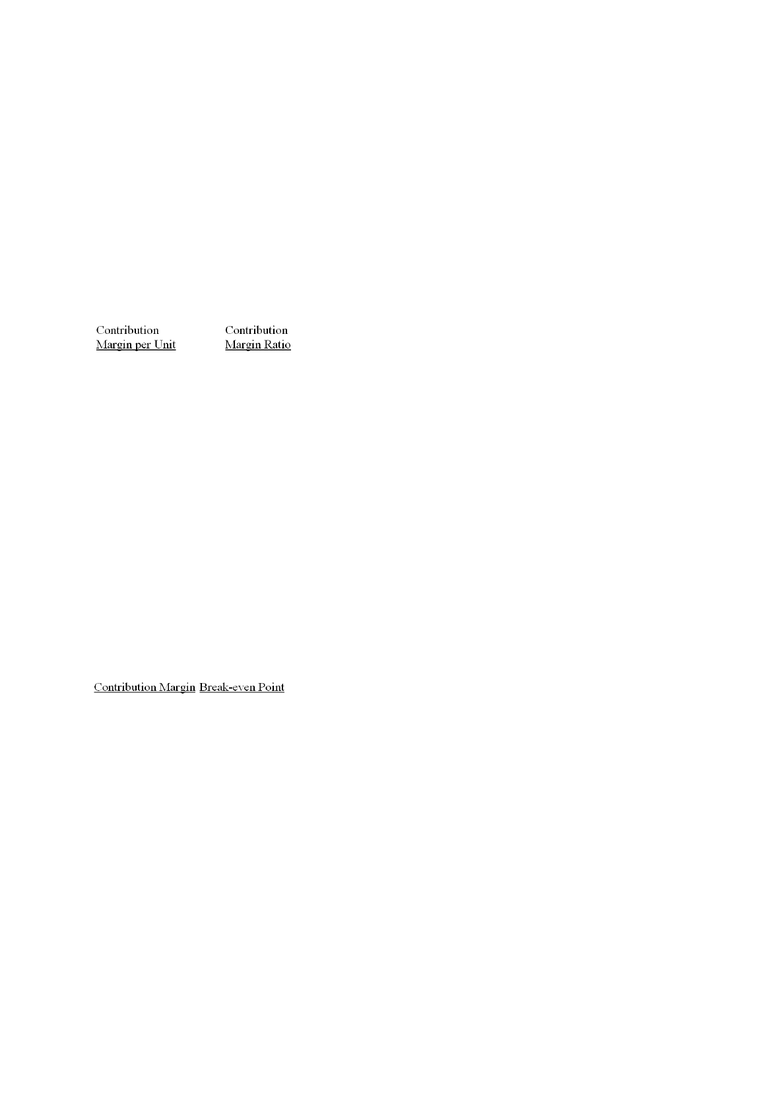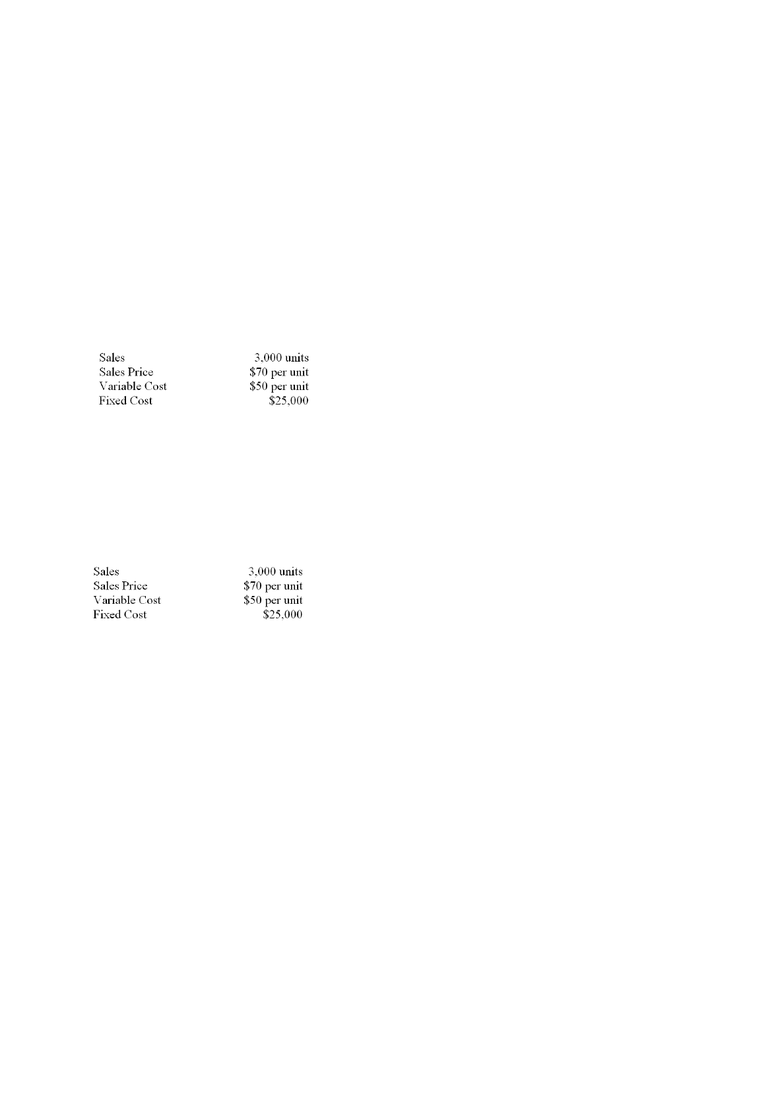Class Notes (1,100,000)
CA (630,000)
York (40,000)
Lecture 7

# ADMS 2510 Lecture 7: ch07.pdf

Department
Course Code
Professor
Alison Beavis
Lecture
7

This preview shows pages 1-3. to view the full 51 pages of the document.ch07
Student: ___________________________________________________________________________
1. Which of the following is defined as the difference between total sales in dollars and total variable
expenses?
A. Margin of safety.
B. Operating income.
C. The gross margin.
D. The contribution margin.
2. Brasher Company manufactures and sells a single product that has a positive contribution margin. If the
selling price and variable expenses both decrease by 5% and fixed expenses do not change, then what
would be the effect on the contribution margin per unit and the contribution margin ratio?
A. Decrease Decrease
B. Decrease No change
C. No change Decrease
D. No change No change
3. Once the break-even point is reached, which of the following statements is true?
A. The total contribution margin changes from negative to positive.
B. Operating income will increase by the unit contribution margin for each additional item sold.
C. Variable expenses will remain constant in total.
D. The contribution margin ratio begins to decrease.
4. The contribution margin ratio always increases when which of the following occurs?
A. Variable expenses as a percentage of sales increase.
B. Variable expenses as a percentage of sales decrease.
C. Break-even point increases.
D. Break-even point decreases.
5. If the fixed expenses of a product increase while variable expenses and the selling price remain constant,
what will happen to the total contribution margin and the break-even point?
A. Increase Decrease
B. Decrease Increase
C. Unchanged Increase
D. Unchanged Unchanged
6. The total contribution margin decreases if sales volume remains the same and which of the following
occurs?
A. Fixed expenses increase.
B. Fixed expenses decrease.
C. Variable expense per unit increases.
D. Variable expense per unit decreases.
7. The break-even in units sold will decrease if there is an increase in which of the following?
A. Unit sales volume.
B. Total fixed expenses.
C. Unit variable expenses.
D. Selling price.

Only pages 1-3 are available for preview. Some parts have been intentionally blurred.8. A company has sales of \$87,500 at the break even point and fixed costs are \$35,000. Assuming cost
behaviour does not change if sales increase by \$20,000 how much will operating income will increase
by?
A. \$20,000.00.
B. \$12,000.00.
C. \$8,000.00.
D. \$4,000.00.
9. A company increased the selling price for its product from \$1.00 to \$1.10 a unit when total fixed
expenses increased from \$400,000 to \$480,000 and the variable expense per unit remained unchanged at
\$0.50. How would these changes affect the break-even point?
A. The break-even point in units would increase.
B. The break-even point in units would decrease.
C. The break-even point in units would remain unchanged.
D. The effect cannot be determined from the information given.
10. Which of the following is defined as the ratio of fixed expenses to the unit contribution margin?
A. Break-even point in unit sales.
B. Profit margin.
C. Contribution margin ratio.
D. Margin of safety.
11. The break-even point in unit sales increases when variable expenses do which of the following?
A. Increase, and the selling price remains unchanged.
B. Decrease, and the selling price remains unchanged.
C. Decrease, and the selling price increases.
D. Remain unchanged, and the selling price increases.
12. How is the margin of safety percentage computed?
A. Break-even sales divided by Total sales.
B. Total sales minus Break-even sales.
C. (Total sales-Break-even sales) divided by Break-even sales.
D. (Total sales-Break-even sales) divided by Total sales.
13. When interpreting a CVP graph which of the following is NOT correct?
A. When sales are below the breakeven intersection the company incurs a loss.
B. The breakeven point is where the total revenue line meets the fixed cost line.
C
. The anticipated profit or loss at any given level of sales is measured by the vertical distance between
the total revenue line and the total expense line.
D. The total revenue line starts at the origin.
14. Which of the following is defined as the amount by which a company's sales can decline before operating
losses are incurred?
A. Contribution margin.
B. Degree of operating leverage.
C. Margin of safety.
D. Contribution margin ratio.
15. How is the degree of operating leverage calculated?
A. Contribution margin divided by sales.
B. Gross margin divided by operating income.
C. Operating income divided by sales.
D. Contribution margin divided by operating income.

Only pages 1-3 are available for preview. Some parts have been intentionally blurred.16. If company A has a higher degree of operating leverage than company B, then which of the following
statements is true?
A. Company A has higher variable expenses.
B. Company A's profits are more sensitive to percentage changes in sales.
C. Company A is more profitable.
D. Company A is less risky.
17. Marston Enterprises sells three chemicals: petrol, septine, and tridol. Petrol's unit contribution margin is
higher than septine's, which is higher than tridol's. Which one of the following events is most likely to
increase the company's overall break-even point?
A.
The installation of new computer-controlled equipment and subsequent lay-off of assembly-line
workers.
B. A decrease in tridol's selling price.
C. An increase in the overall market demand for septine.
D.
A change in the relative market demand for the products, with the increase favouring petrol relative to
septine and tridol.
18. A company has provided the following data:
If the dollar contribution margin per unit is increased by 10%, total fixed cost is decreased by 20%, and
all other factors remain the same, what will the outcome be for operating income?
A. Increase by \$61,000.
B. Increase by \$20,000.
C. Increase by \$3,500.
D. Increase by \$11,000.
19. A company has provided the following data:
If the sales volume decreases by 25%, the variable cost per unit increases by 15%, and all other factors
remain the same, what will the outcome be for operating income?
A. Decrease by \$31,875.
B. Decrease by \$15,000.
C. Increase by \$20,625.
D. Decrease by \$3,125.
20. Last year, Twins Company reported \$750,000 in sales (25,000 units) and an operating income of
\$25,000. At the break-even point, the company's total contribution margin equals \$500,000. Based on this
information, which of the following statements is true?
A. The company's contribution margin ratio is 40%.
B. The company's break-even point is 24,000 units.
C. The company's variable expense per unit is \$9.
D. The company's variable expenses are 60% of sales.
21. Last year, Black Company reported sales of \$640,000, a contribution margin of \$160,000, and an
operating loss of \$40,000. Based on this information, what was the break-even point?
A. \$640,000.
B. \$480,000.
C. \$800,000.
D. \$960,000.